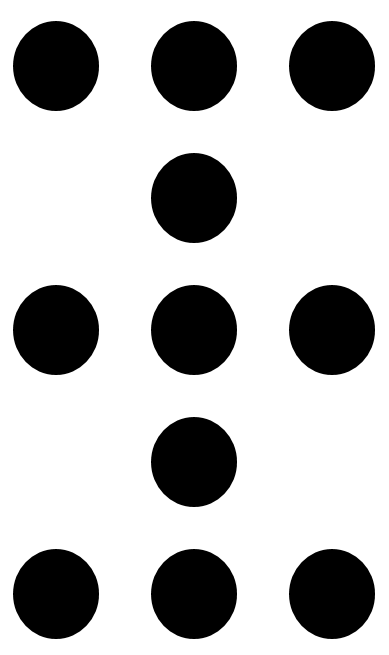# Dot Card Counting

Directions: How can we figure out how many dots there are below?### Hint

What is another strategy for figuring out the number of dots?

Strategies will vary for counting the 11 dots.  Some will see a column of 5 down the middle and 2 sets of 3 dots on either side.  Some will see 3 rows of 3 dots and 2 extra dots between them.

Source: Dan Meyer.  Additional examples can be found here using a resource provided by Melissa Canham.

### Check Also

Directions: Using the digits 0 to 9 at most one time each, place a digit …

1.The next step in dot card counting is moving kids to think about equivalence. Using the above sample, I’ve turned to first graders and asked them, “Is is legal to say, 5+3+3 = 2+3+3+3? Most will say, “No!” But a few will add up both sides and find that each side equals 11. And then, there’s always one or two who combine the 2 with a 3 on the right side of the equation and prove equivalency by showing 5+3+3 = 5+3+3.

2.An ‘interesting’ question for students is how they’d count the same card if it were rotated 90 degrees. I tried it and the strategies changed for most students. They see the ‘pattern’ differently depending on the orientation. Of course, this can be used to introduce, informally, the idea of commutativity.

3.Love this sort of problem, have invented many myself. Where this goes next, for me, is to ask how would you animate the dots to a new configuration to make counting simpler?

4.I did 3+3+3+2=11

5.i put them into parts so it would be easy to count them, i knew that 3+3+3=9 and 9+2= 11

6.7.Yes, I agree that would be an excellent idea. It would really help them to not be so static in their thinking.

8.MARY SUTHERLAND

We should move from who can get the answer first, to how many different ways could you look at it.

9.10.Hey there just wanted to give you a quick heads up and let you know a few of the pictures aren’t loading properly.
I’m not sure why but I think its a linking issue. I’ve tried it in two different internet browsers and both show the same results.